# Bar Plots and Modern Alternatives

June 28, 2017
By

Want to share your content on R-bloggers? click here if you have a blog, or here if you don't.

This article describes how to create easily basic and ordered bar plots using ggplot2 based helper functions available in the ggpubr R package. We’ll also present some modern alternatives to bar plots, including lollipop charts and cleveland’s dot plots.

Note that, the approach to build a bar plot, using ggplot2 standard verbs, has been described in our previous article available at: ggplot2 barplots : Quick start guide.

You might be also interested by the following articles: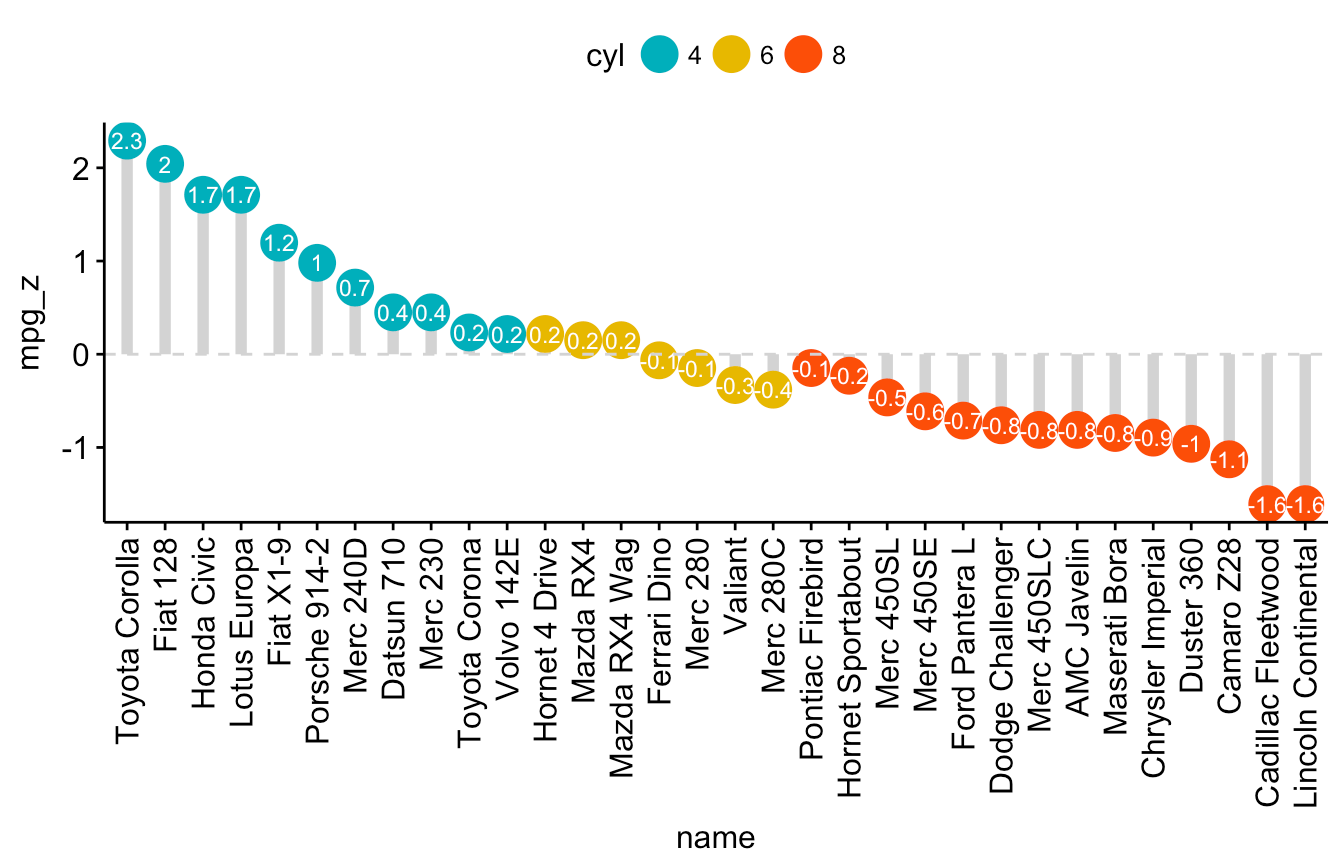Bar plots and modern alternatives

Contents:

## Prerequisites

### Required R package

You need to install the R package ggpubr (version >= 0.1.3), to easily create ggplot2-based publication ready plots.

Install from CRAN:

``install.packages("ggpubr")``

Or, install the latest developmental version from GitHub as follow:

``````if(!require(devtools)) install.packages("devtools")
devtools::install_github("kassambara/ggpubr")``````

``library(ggpubr)``

## Basic bar plots

Create a demo data set:

``````df <- data.frame(dose=c("D0.5", "D1", "D2"),
len=c(4.2, 10, 29.5))
print(df)``````
``````  dose  len
1 D0.5  4.2
2   D1 10.0
3   D2 29.5``````

Basic bar plots:

``````# Basic bar plots with label
p <- ggbarplot(df, x = "dose", y = "len",
color = "black", fill = "lightgray")
p

# Rotate to create horizontal bar plots
p + rotate()``````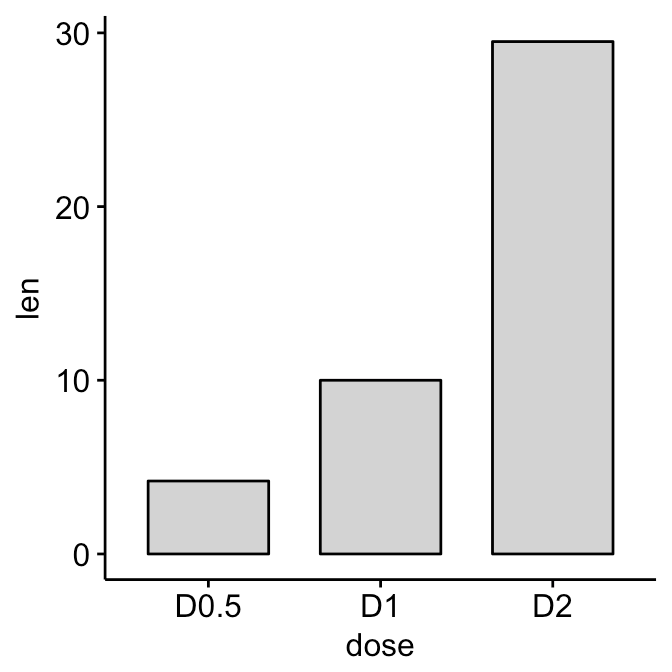Bar plots and modern alternatives

Change fill and outline colors by groups:

``````ggbarplot(df, x = "dose", y = "len",
fill = "dose", color = "dose", palette = "jco")``````Bar plots and modern alternatives

## Multiple grouping variables

Create a demo data set:

``````df2 <- data.frame(supp=rep(c("VC", "OJ"), each=3),
dose=rep(c("D0.5", "D1", "D2"),2),
len=c(6.8, 15, 33, 4.2, 10, 29.5))
print(df2)``````
``````  supp dose  len
1   VC D0.5  6.8
2   VC   D1 15.0
3   VC   D2 33.0
4   OJ D0.5  4.2
5   OJ   D1 10.0
6   OJ   D2 29.5``````

Plot y = “len” by x = “dose” and change color by a second group: “supp”

``````# Stacked bar plots, add labels inside bars
ggbarplot(df2, x = "dose", y = "len",
fill = "supp", color = "supp",
palette = c("gray", "black"),
label = TRUE, lab.col = "white", lab.pos = "in")

# Change position: Interleaved (dodged) bar plot
ggbarplot(df2, x = "dose", y = "len",
fill = "supp", color = "supp",
palette = c("gray", "black"),
position = position_dodge(0.9))``````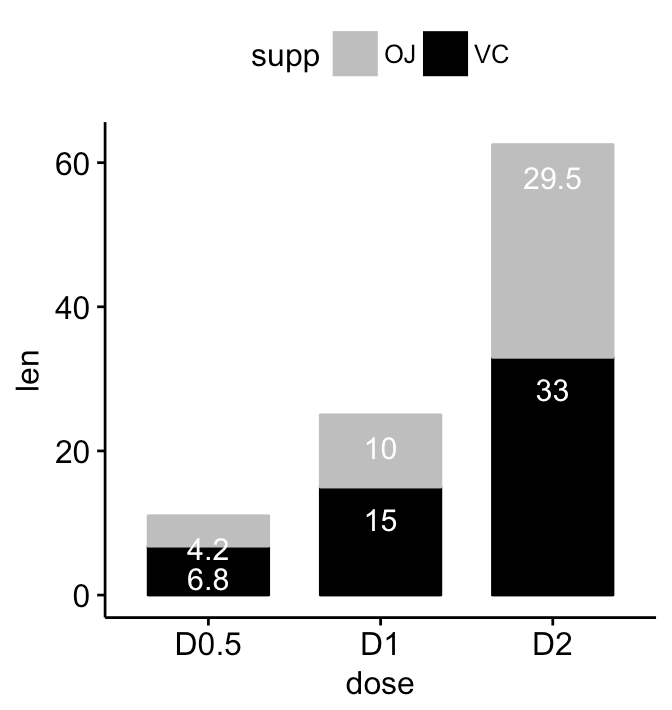Bar plots and modern alternatives

## Ordered bar plots

``````# Load data
data("mtcars")
dfm <- mtcars
# Convert the cyl variable to a factor
dfm\$cyl <- as.factor(dfm\$cyl)
dfm\$name <- rownames(dfm)
# Inspect the data
``````                               name    wt  mpg cyl
Mazda RX4                 Mazda RX4 2.620 21.0   6
Mazda RX4 Wag         Mazda RX4 Wag 2.875 21.0   6
Datsun 710               Datsun 710 2.320 22.8   4
Hornet 4 Drive       Hornet 4 Drive 3.215 21.4   6
Valiant                     Valiant 3.460 18.1   6``````

Create ordered bar plots. Change the fill color by the grouping variable “cyl”. Sorting will be done globally, but not by groups.

``````ggbarplot(dfm, x = "name", y = "mpg",
fill = "cyl",               # change fill color by cyl
color = "white",            # Set bar border colors to white
palette = "jco",            # jco journal color palett. see ?ggpar
sort.val = "desc",          # Sort the value in dscending order
sort.by.groups = FALSE,     # Don't sort inside each group
x.text.angle = 90           # Rotate vertically x axis texts
)``````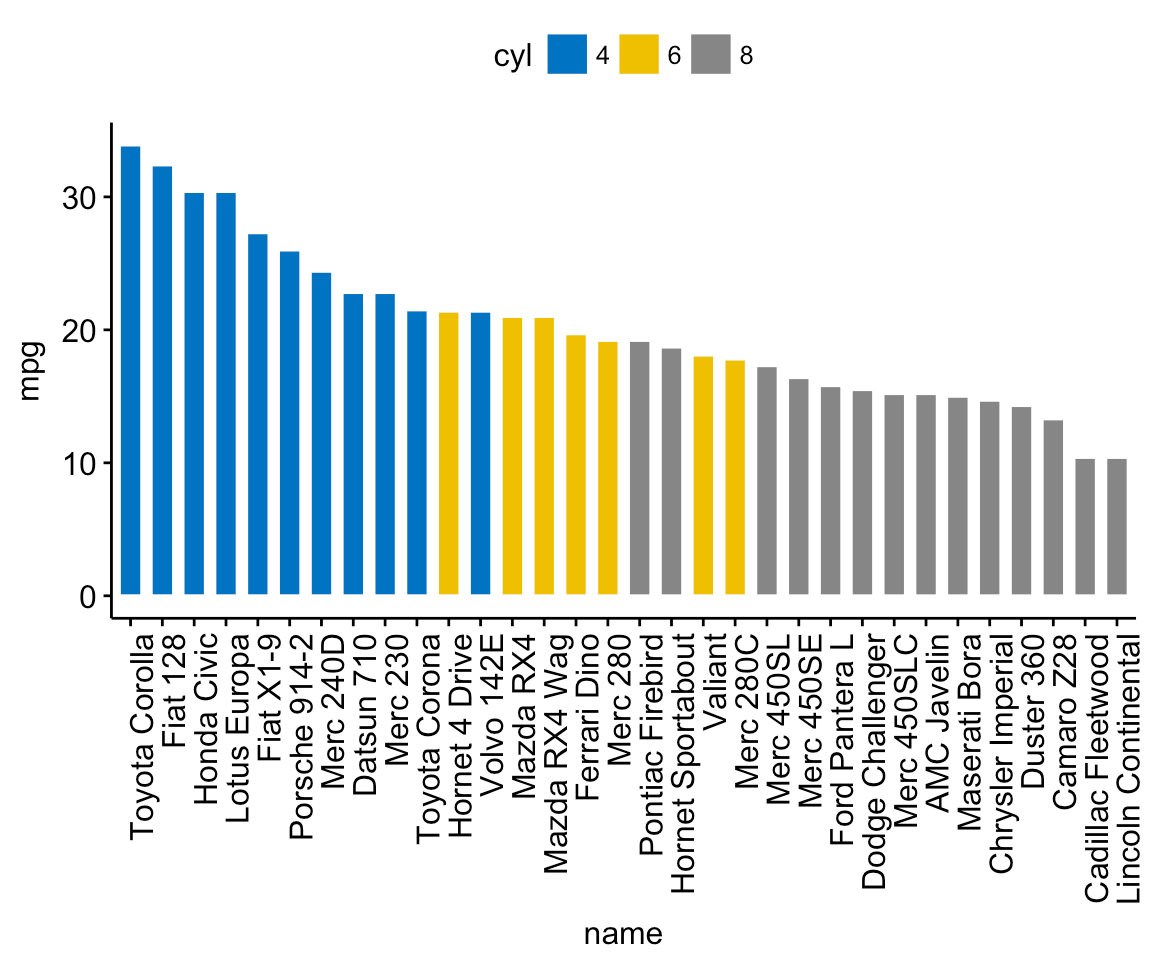Bar plots and modern alternatives

Sort bars inside each group. Use the argument sort.by.groups = TRUE.

``````ggbarplot(dfm, x = "name", y = "mpg",
fill = "cyl",               # change fill color by cyl
color = "white",            # Set bar border colors to white
palette = "jco",            # jco journal color palett. see ?ggpar
sort.val = "asc",           # Sort the value in dscending order
sort.by.groups = TRUE,      # Sort inside each group
x.text.angle = 90           # Rotate vertically x axis texts
)``````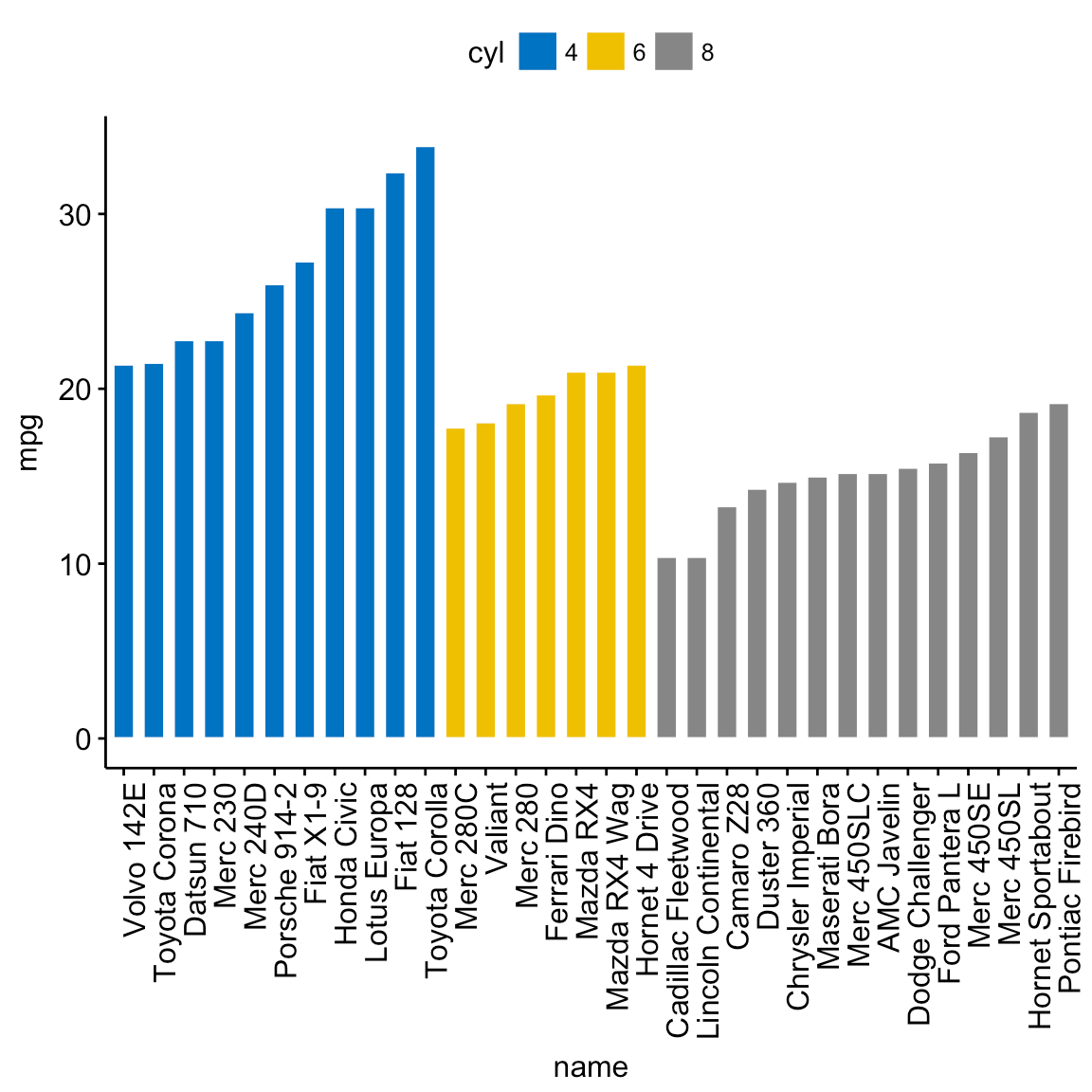Bar plots and modern alternatives

## Deviation graphs

The deviation graph shows the deviation of quantitative values to a reference value. In the R code below, we’ll plot the mpg z-score from the mtcars data set.

Calculate the z-score of the mpg data:

``````# Calculate the z-score of the mpg data
dfm\$mpg_z <- (dfm\$mpg -mean(dfm\$mpg))/sd(dfm\$mpg)
dfm\$mpg_grp <- factor(ifelse(dfm\$mpg_z < 0, "low", "high"),
levels = c("low", "high"))
# Inspect the data
head(dfm[, c("name", "wt", "mpg", "mpg_z", "mpg_grp", "cyl")])``````
``````                               name    wt  mpg      mpg_z mpg_grp cyl
Mazda RX4                 Mazda RX4 2.620 21.0  0.1508848    high   6
Mazda RX4 Wag         Mazda RX4 Wag 2.875 21.0  0.1508848    high   6
Datsun 710               Datsun 710 2.320 22.8  0.4495434    high   4
Hornet 4 Drive       Hornet 4 Drive 3.215 21.4  0.2172534    high   6
Valiant                     Valiant 3.460 18.1 -0.3302874     low   6``````

Create an ordered bar plot, colored according to the level of mpg:

``````ggbarplot(dfm, x = "name", y = "mpg_z",
fill = "mpg_grp",           # change fill color by mpg_level
color = "white",            # Set bar border colors to white
palette = "jco",            # jco journal color palett. see ?ggpar
sort.val = "asc",           # Sort the value in ascending order
sort.by.groups = FALSE,     # Don't sort inside each group
x.text.angle = 90,          # Rotate vertically x axis texts
ylab = "MPG z-score",
xlab = FALSE,
legend.title = "MPG Group"
)``````Bar plots and modern alternatives

Rotate the plot: use rotate = TRUE and sort.val = “desc”

``````ggbarplot(dfm, x = "name", y = "mpg_z",
fill = "mpg_grp",           # change fill color by mpg_level
color = "white",            # Set bar border colors to white
palette = "jco",            # jco journal color palett. see ?ggpar
sort.val = "desc",          # Sort the value in descending order
sort.by.groups = FALSE,     # Don't sort inside each group
x.text.angle = 90,          # Rotate vertically x axis texts
ylab = "MPG z-score",
legend.title = "MPG Group",
rotate = TRUE,
ggtheme = theme_minimal()
)``````Bar plots and modern alternatives

## Alternatives to bar plots

### Lollipop chart

Lollipop chart is an alternative to bar plots, when you have a large set of values to visualize.

Lollipop chart colored by the grouping variable “cyl”:

``````ggdotchart(dfm, x = "name", y = "mpg",
color = "cyl",                                # Color by groups
palette = c("#00AFBB", "#E7B800", "#FC4E07"), # Custom color palette
sorting = "ascending",                        # Sort value in descending order
add = "segments",                             # Add segments from y = 0 to dots
ggtheme = theme_pubr()                        # ggplot2 theme
)``````Bar plots and modern alternatives

• Sort in descending order. sorting = “descending”.
• Rotate the plot vertically, using rotate = TRUE.
• Sort the mpg value inside each group by using group = “cyl”.
• Set dot.size to 6.
• Add mpg values as label. label = “mpg” or label = round(dfm\$mpg).
``````ggdotchart(dfm, x = "name", y = "mpg",
color = "cyl",                                # Color by groups
palette = c("#00AFBB", "#E7B800", "#FC4E07"), # Custom color palette
sorting = "descending",                       # Sort value in descending order
add = "segments",                             # Add segments from y = 0 to dots
rotate = TRUE,                                # Rotate vertically
group = "cyl",                                # Order by groups
dot.size = 6,                                 # Large dot size
label = round(dfm\$mpg),                        # Add mpg values as dot labels
font.label = list(color = "white", size = 9,
vjust = 0.5),               # Adjust label parameters
ggtheme = theme_pubr()                        # ggplot2 theme
)``````Bar plots and modern alternatives

Deviation graph:

• Use y = “mpg_z”
• Change segment color and size: add.params = list(color = “lightgray”, size = 2)
``````ggdotchart(dfm, x = "name", y = "mpg_z",
color = "cyl",                                # Color by groups
palette = c("#00AFBB", "#E7B800", "#FC4E07"), # Custom color palette
sorting = "descending",                       # Sort value in descending order
add = "segments",                             # Add segments from y = 0 to dots
add.params = list(color = "lightgray", size = 2), # Change segment color and size
group = "cyl",                                # Order by groups
dot.size = 6,                                 # Large dot size
label = round(dfm\$mpg_z,1),                        # Add mpg values as dot labels
font.label = list(color = "white", size = 9,
vjust = 0.5),               # Adjust label parameters
ggtheme = theme_pubr()                        # ggplot2 theme
)+
geom_hline(yintercept = 0, linetype = 2, color = "lightgray")``````Bar plots and modern alternatives

### Cleveland’s dot plot

Color y text by groups. Use y.text.col = TRUE.

``````ggdotchart(dfm, x = "name", y = "mpg",
color = "cyl",                                # Color by groups
palette = c("#00AFBB", "#E7B800", "#FC4E07"), # Custom color palette
sorting = "descending",                       # Sort value in descending order
rotate = TRUE,                                # Rotate vertically
dot.size = 2,                                 # Large dot size
y.text.col = TRUE,                            # Color y text by groups
ggtheme = theme_pubr()                        # ggplot2 theme
)+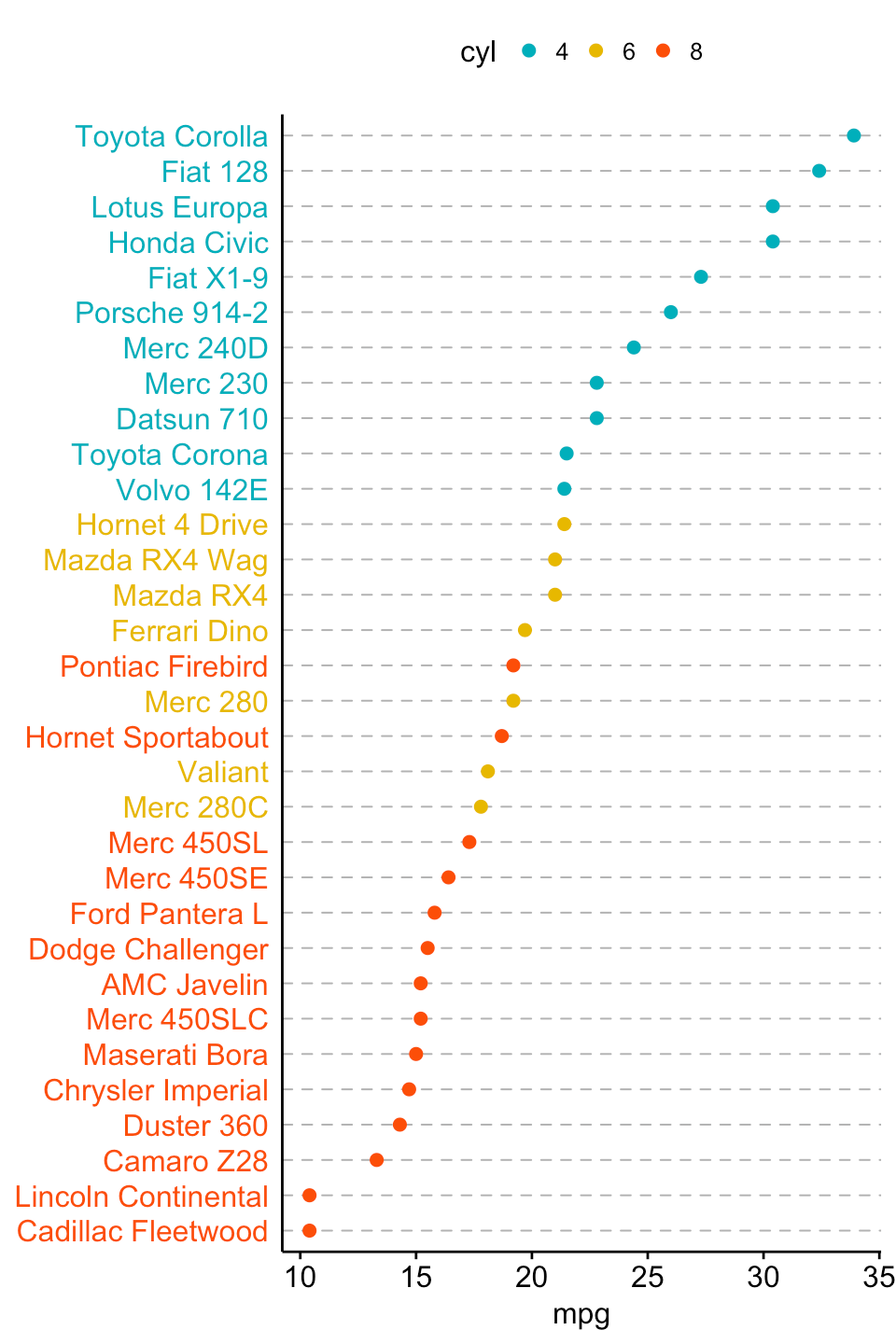Bar plots and modern alternatives

## Infos

This analysis has been performed using R software (ver. 3.3.2) and ggpubr (ver. 0.1.4).

R-bloggers.com offers daily e-mail updates about R news and tutorials about learning R and many other topics. Click here if you're looking to post or find an R/data-science job.
Want to share your content on R-bloggers? click here if you have a blog, or here if you don't.